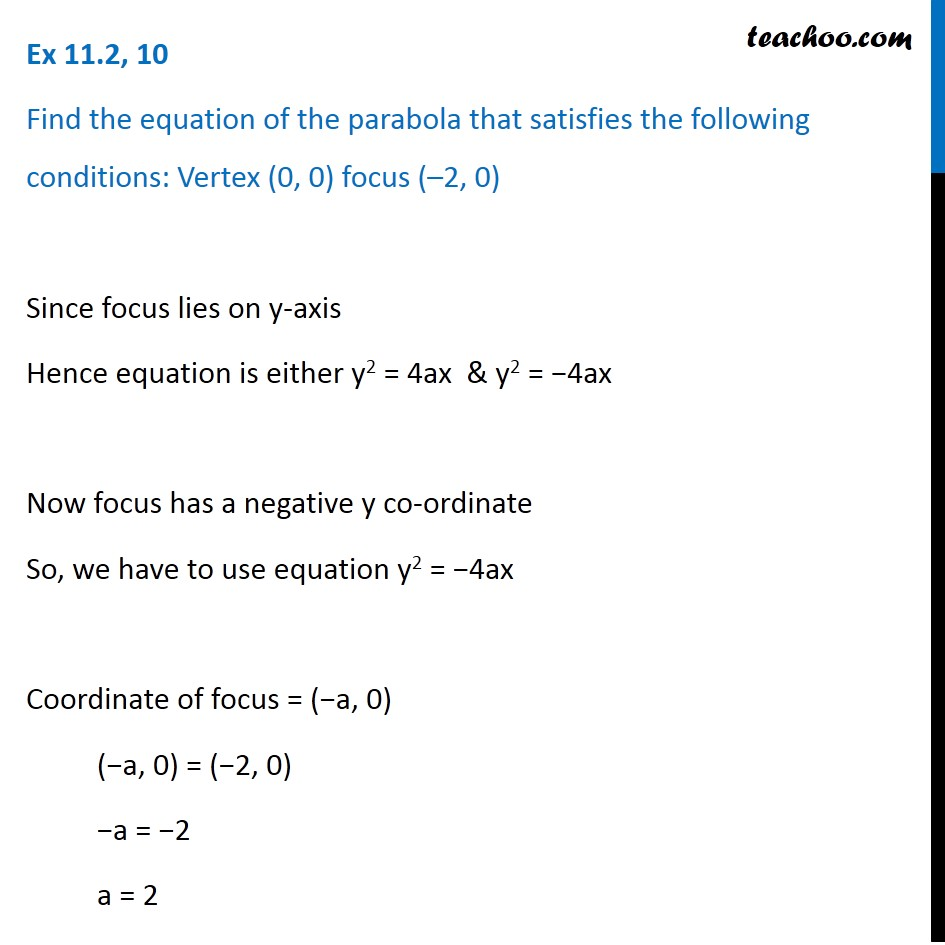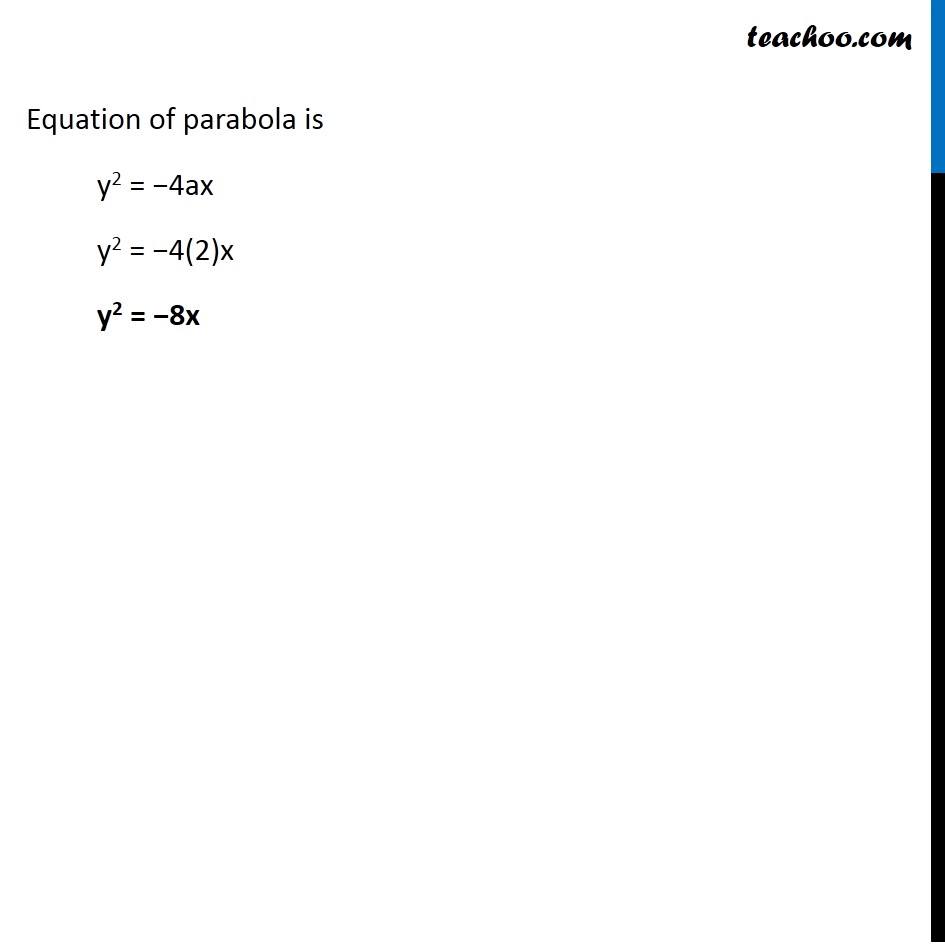Ex 10.2

Chapter 10 Class 11 Conic Sections
Serial order wiseLearn in your speed, with individual attention - Teachoo Maths 1-on-1 Class

### Transcript

Ex 10.2, 10 Find the equation of the parabola that satisfies the following conditions: Vertex (0, 0) focus (–2, 0) Since focus lies on y-axis Hence equation is either y2 = 4ax & y2 = −4ax Now focus has a negative y co-ordinate So, we have to use equation y2 = −4ax Coordinate of focus = (−a, 0) (−a, 0) = (−2, 0) −a = −2 a = 2 Equation of parabola is y2 = −4ax y2 = −4(2)x y2 = −8x